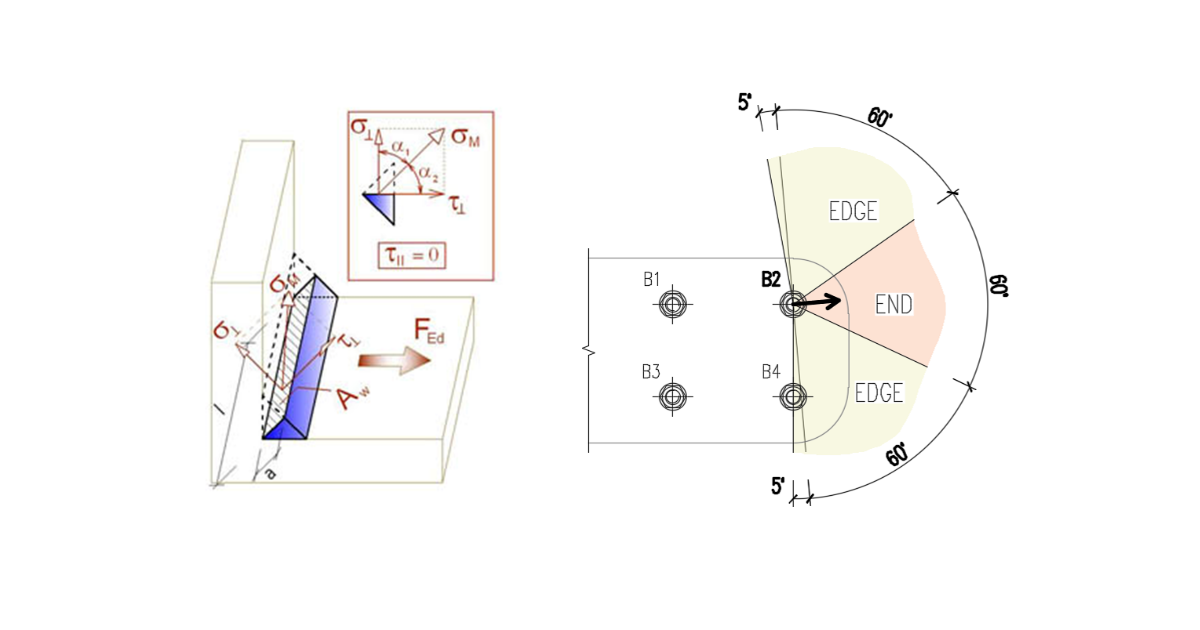### Válasszon nyelvet,# Detailing of bolts and welds according to Chinese standard

SupportCenter.LocalizedArticle
$$BoltsThe minimum allowable distance of bolts is checked according to Table 11.5.2.Minimum allowable distance of bolts; d0 – bolt hole diameter Minimum allowable distance Bolt pitch$$3 \cdot d_0$$ End distance parallel to load$$2 \cdot d_0$$ Edge distance perpendicular to load (bearing bolt type) $$1.2 \cdot d_0$$ Edge distance perpendicular to load (preloded bolt type)$$1.5 \cdot d_0$$ WeldsThe minimal weld size hf is checked according to Table 11.3.5. The weld size is determined from weld throat thickness: $$h_f = \sqrt{2} \cdot h_e$$.Minimal weld size hf Thickness of plate [mm]Minimum weld size [mm] $$t \le 6$$3 $$6 < t \le 12$$5 $$12 < t \le 20$$ 6 \( 20